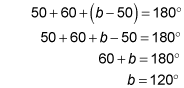##### Geometry: 1,001 Practice Problems For Dummies (+ Free Online Practice)In geometry, you can use the exterior angle of a triangle to find a missing interior angle. The following practice questions ask you to do just that, and then to apply some algebra, along with the properties of an exterior angle, to find a missing variable.

## Practice questions

Use the figure and the given information to answer the following questions.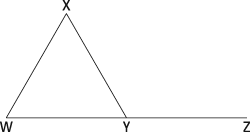1. In triangle WXY,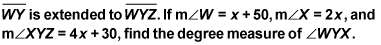2. In triangle WXY,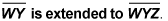Each angle is represented by the following: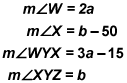Solve for the variable b.

1. 70 degrees

The exterior angle of a triangle is equal to the sum of the two nonadjacent interior angles of the triangle, so set up an equation and solve for x: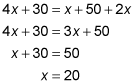Plug in the value for x to find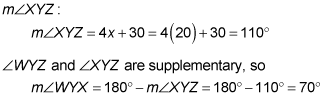2. 120 degrees

The exterior angle of a triangle is equal to the sum of the two nonadjacent interior angles of the triangle. Use this relationship to solve for a: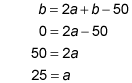Plug in the value of a to find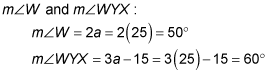The angles of a triangle add up to 180 degrees. Therefore,